# Derive the Power Relation for Single Tone AM Wave and Multiple-tone AM Wave

## Definition

It may be observed from the expression of AM wave that the carrier component of the amplitude modulated wave has the same amplitude as unmodulated carrier.

In addition to carrier component , the modulated wave consists of two sideband components. It means that the modulated wave contains more power than the unmodulated carrier.

However, since the amplitudes of two sidebands depend upon the modulation index, it may be anticipated that the total power of the amplitude modulated wave would depend upon the modulation index also. In this section, we shall find the power contents of the carrier and the sidebands.

As we know  the general expression of AM wave is given as :

s(t) = A cos ωct + x(t) cos ωct       ………………..(1)

The total power P of the AM wave is the sum of the carrier power PC and sideband power PS.

## Carrier Power

The carrier power PC is equal to the mean-square (ms) value of the carrier term A cos ωct i.e.

PC =  mean square value of A cos ωct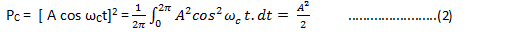## Sideband Power

The sideband power PS is equal to the mean square value of the sideband term x(t) cos ωct i.e.

PS =  mean square value of x(t) cos ωctIn AM generation , a Band pass filter (BPF) or a tuned circuit tuned to carrier frequency ωc is used to filter out the second integral term.

Therefore,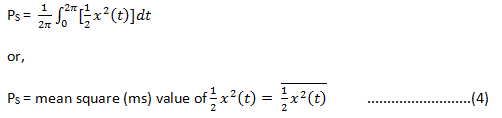However, the total sideband power PS is due to the equal contributions of the upper and lower sidebands. Hence, the power carried by the upper and lower sidebands will be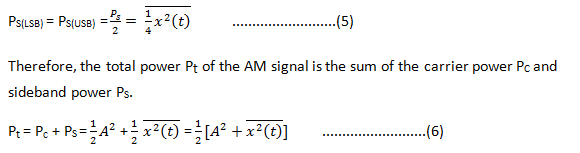## Power of a Single-Tone Amplitude-Modulated (AM) Signal

### Definition

We know that the power content of the AM signal when modulating-signal is a random signal and may consist of several frequency components. Likewise we can find power content of single-tone Amplitude Modulated (AM) signal.

### Mathematical Expression

Let us consider that a carrier signal A cos ωct is amplitude-modulated by a single-tone modulating signal x(t) =Vm A cos ωmt.

Then the unmodulated or carrier power

PC =  mean square (ms value)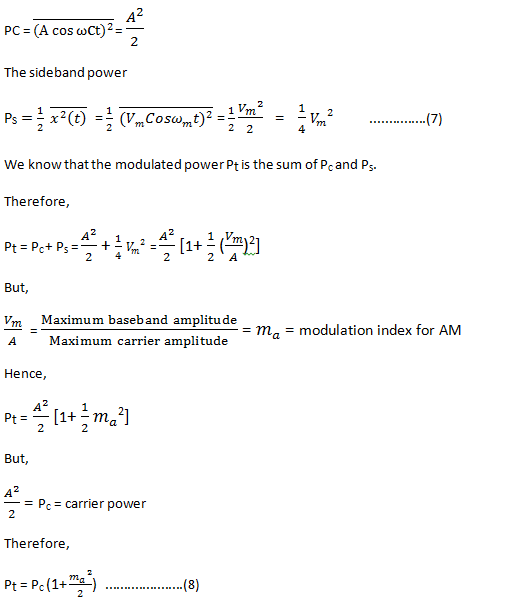## Power Content In Multiple-tone Amplitude Modulation (AM)

### Definition

A multiple-tone amplitude modulation is that type of modulation in which the modulating signal consists of more than one frequency components.

### Mathematical Expression

Let us consider that a carrier signal A cos ωct is modulated by a baseband or modulating signal x(t) which is expressed as :

x(t) = V1 cos ω1t + V2 cos ω2t + V3 cos ω3t          ………………….(9)

We know that the general expression for AM wave is

s(t) = A cos ωct + x(t) cos ωct       ………………..(10)

Putting the value of x(t), we get

s(t) = A cos ωct + [V1 cos ω1t + V2 cos ω2t + V3 cos ω3t ] cos ωct

or,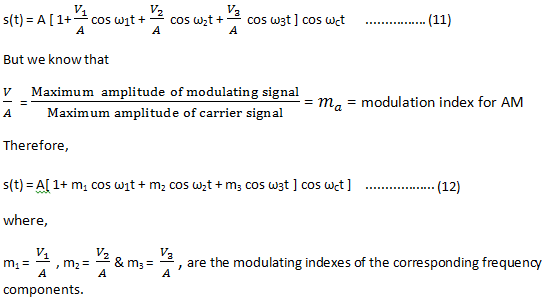The expression for AM wave in equation (12) can further be expanded as under:

s(t) = A cos ωct + m1 A cos ωct cos ω1t + m2 A cos ωct cos ω2t + m3 A cos ωct cos ω3t

Now we know that the total power in AM is given as

Pt = carrier power + sideband power

Pt = PC + PS …………(13)

The carrier power PC is given as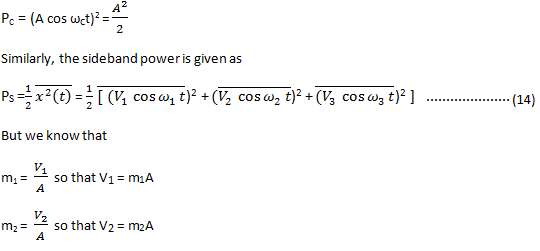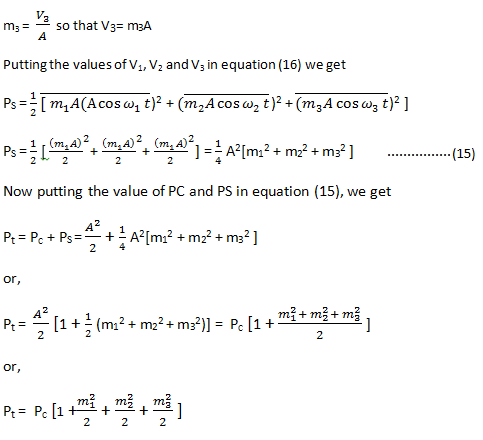## Total or Net Modulation Index for Multiple-Tone Modulation

### Definition

Let us consider that mt is the total or net modulation indexes for a multiple-tone modulation.

### Mathematical Expression

We know that for a multiple-tone modulation, the total power is expressed as :Where, m1 , m2 , …… mn are the modulation indexes for different modulating signals.

The power for AM wave is also expressed as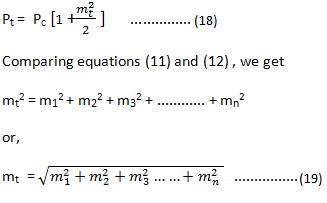This is the desired expression for the total or net modulation index.